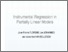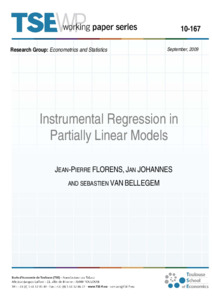# Instrumental Regression in Partially Linear Models

Florens, Jean-Pierre, Johannes, Jan and Van Bellegem, Sébastien (2009) Instrumental Regression in Partially Linear Models. TSE Working Paper, n. 10-167Preview
Text
Official URL: http://tse-fr.eu/pub/22820

## Abstract

We consider the semiparametric regression Xtβ+φ(Z) where β and φ(·) are unknown slope coefficient vector and function, and where the variables (X,Z) are endogeneous. We propose necessary and sufficient conditions for the identification of the parameters
in the presence of instrumental variables. We also focus on the estimation of β. An incorrect parameterization of φ may generally lead to an inconsistent estimator of β, whereas even consistent nonparametric estimators for φ imply a slow rate of convergence
of the estimator of β. An additional complication is that the solution of the equation necessitates the inversion of a compact operator that has to be estimated nonparametrically. In general this inversion is not stable, thus the estimation of β is ill-posed. In this paper, a √n-consistent estimator for β is derived under mild assumptions. One of these assumptions is given by the so-called source condition that is explicitly interprated in the paper. Finally we show that the estimator achieves the semiparametric efficiency bound, even if the model is heteroscedastic. Monte Carlo simulations demonstrate the reasonable performance of the estimation procedure on finite samples.

Item Type: Monograph (Working Paper) English September 2009 C14 - Semiparametric and Nonparametric MethodsC30 - General B- ECONOMIE ET FINANCE TSE-R (Toulouse) UT1 18 Jan 2012 06:02 07 Mar 2018 13:22 oai:tse-fr.eu:22820 http://publications.ut-capitole.fr/id/eprint/3386View Item